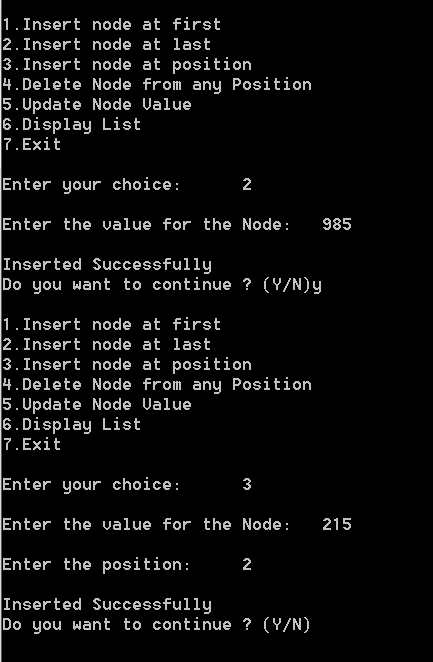## Tuesday, 23 February 2016

### Data Structures - Cpp Program to implement Linked List Operations - Insert , Delete , Update , Display

Linked List is another data structure consisting of group of nodes which together represent a sequence. Each node has two parts one for the value and other for link to the next node.
Linked lists allows dynamic memory allocation.A singly linked list whose nodes contain two fields: an integer value and a link to the next node

 Some of the operations in a linked list are : Insertion into the list Deletion from the list Updation of the value Display the list

Download code

## PROGRAM :

```        #include<iostream>
#include<cstdio>
#include<cstdlib>

using namespace std;

struct node
{
int value;
struct node *next;
}*start;

typedef struct node snode;
snode *newnode, *ptr, *prev, *temp;
snode *first = NULL, *last = NULL;

class LinkedList
{
public:
node* create_node(int);
void insertNodeFirst();
void insertNodeLast();
void insertNodePosition();
void deleteNode();
void updateNode();
void displayList();
LinkedList()
{
start = NULL;
}
};

int main()
{
int ch;
char ans = 'Y';
LinkedList ll;
while (ans == 'Y'||ans == 'y')
{
cout << "\n1.Insert node at first";
cout << "\n2.Insert node at last";
cout << "\n3.Insert node at position";
cout << "\n4.Delete Node from any Position";
cout << "\n5.Update Node Value";
cout << "\n6.Display List";
cout << "\n7.Exit\n";
cout << "\nEnter your choice: \t";
cin >> ch;

switch (ch)
{
case 1:
ll.insertNodeFirst();
break;
case 2:
ll.insertNodeLast();
break;
case 3:
ll.insertNodePosition();
break;
case 4:
ll.deleteNode();
break;
case 5:
ll.updateNode();
break;
case 6:
ll.displayList();
break;
case 7:
return 0;
break;
default:
cout << "\nInvalid Choice\n";
break;
}
cout << "\nDo you want to continue ? (Y/N) \t";
cin >> ans;
}
return 0;
}
snode* createNode(int val)
{
newnode = (snode *)malloc(sizeof(snode));
if (newnode == NULL)
{
cout << "\nMemory was not allocated";
return 0;
}
else
{
newnode->value = val;
newnode->next = NULL;
return newnode;
}
}
void LinkedList::insertNodeFirst()
{
int val;
cout << "\nEnter the value for the node: \t";
cin >> val;
newnode = createNode(val);
if (first == last && first == NULL)
{
first = last = newnode;
first->next = NULL;
last->next = NULL;
}
else
{
temp = first;
first = newnode;
first->next = temp;
}
cout << "\nInserted Successfully";
}
void LinkedList::insertNodeLast()
{
int val;

cout << "\nEnter the value for the Node: \t";
cin >> val;
newnode = createNode(val);
if (first == last && last == NULL)
{
first = last = newnode;
first->next = NULL;
last->next = NULL;
}
else
{
last->next = newnode;
last = newnode;
last->next = NULL;
}
cout << "\nInserted Successfully";
}
void LinkedList::insertNodePosition()
{
int pos, val, count = 0, i;
cout << "\nEnter the value for the Node: \t";
cin >> val;
newnode = createNode(val);
cout << "\nEnter the position: \t";
cin >> pos;
ptr = first;
while (ptr != NULL)
{
ptr = ptr->next;
count++;
}
if (pos == 1)
{
if (first == last && first == NULL)
{
first = last = newnode;
first->next = NULL;
last->next = NULL;
}
else
{
temp = first;
first = newnode;
first->next = temp;
}
cout << "\nInserted Successfully";
}
else if (pos>1 && pos<=count)
{
ptr = first;
for (i = 1; i < pos; i++)
{
prev = ptr;
ptr = ptr->next;
}
prev->next = newnode;
newnode->next = ptr;
cout << "\nInserted Successfully";
}
else
{
cout << "Position is out of range";
}
}
void LinkedList::deleteNode()
{
int pos, count = 0, i;

if (first == NULL)
{
cout << "No nodes in the list to delete\n";
}
else
{
cout << "\nEnter the position of value to be deleted: \t";
cin >> pos;
ptr = first;
if (pos == 1)
{
first = ptr->next;
cout << "\nElement deleted successfully";
}
else
{
while (ptr != NULL)
{
ptr = ptr->next;
count = count + 1;
}
if (pos > 0 && pos <= count)
{
ptr = first;
for (i = 1; i < pos; i++)
{
prev = ptr;
ptr = ptr->next;
}
prev->next = ptr->next;
}
else
{
cout << "Position is out of range";
}
free(ptr);
cout << "\nElement deleted successfully";
}
}
}
void LinkedList::updateNode()
{
int oldval, newval, flag = 0;

if (first == NULL)
{
cout << "No nodes in the list to update\n";
}
else
{
cout << "\nEnter the value to be updated: \t";
cin >> oldval;
cout << "\nEnter the new value: \t";
cin >> newval;
for (ptr = first; ptr != NULL; ptr = ptr->next)
{
if (ptr->value == oldval)
{
ptr->value = newval;
flag = 1;
break;
}
}
if (flag == 1)
{
cout << "\nUpdated Successfully";
}
else
{
cout << "\nValue not found in List";
}
}
}
void LinkedList::displayList()
{
if (first == NULL)
{
cout << "No nodes in the list to display\n";
}
else
{
for (ptr = first; ptr != NULL; ptr = ptr->next)
{
cout <<  ptr->value << "\t";
}
}
}
```
Download code

## OUTPUT :Cpp - Linked List Operations - Insertion at frontCpp - Linked List Operations - Insertion at last and  any positionCpp - Linked List Operations -  Deletion of nodeCpp - Linked List Operations - Updation of valueCpp - Linked List Operations - Display List
Download code